In Number Bases - Binary, we saw that there are different number base systems, and we looked at how we can convert between binary (base 2) and decimal (base 10). One thing that you may have very quickly worked out, is that converting between binary and decimal isn’t very nice… but writing numbers using binary is long and tedious! This is where hexadecimal comes in.

Hexadecimal is a base 16 number system. This means that the column headers now go up in powers of 16.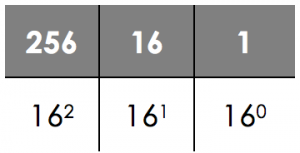In computer science though, we only ever really use 2 digits.

Hexadecimal is used to make binary numbers easier to read for humans, avoid mistakes and allow us to quickly convert into binary. It is important to remember though that computers don’t understand hexadecimal any more than they can understand decimal. It’s just a way for humans to represent numbers.

In hexadecimal, because we now have 16 characters to contend with, we have to add a few to our repertoire. In hexadecimal, the numbers 10-15 are represented using the characters A-F, so the number B in hexadecimal would be 11 in decimal.

Converting between hexadecimal and binary is simple as all we need to worry about is converting each nibble at a time.

Converting between hexadecimal and binary is simple as all we need to worry about is converting each nibble at a time.

To convert a binary number to hexadecimal you need to follow these steps:

1. Split the binary string up into nibbles (remember a nibble = 4 bits)
2. Work out the decimal value for each nibble
3. Convert the decimal value into it’s hex equivalent
4. String together the hex values

Example - Convert 1010 1100 to hexadecimal

First we convert the number into nibbles: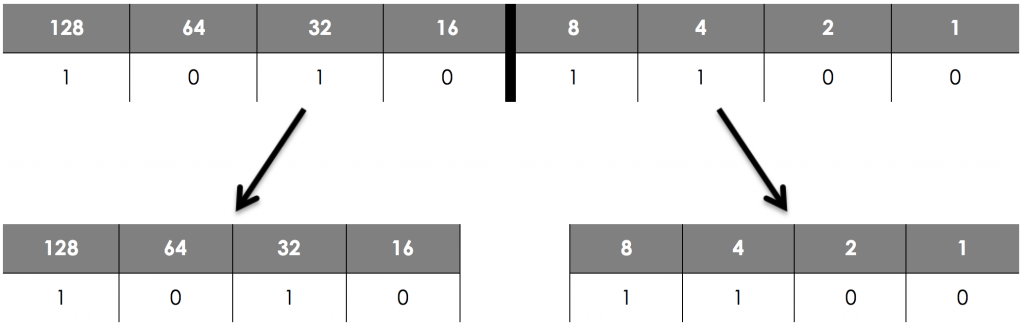Then, looking at each nibble, we convert that into its corresponding hexadecimal digit: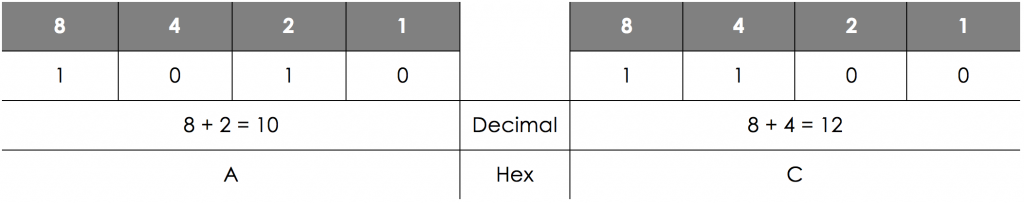So 1010 1100 in Hex is AC.

This is very similar to what we have just seen but the other way around:

1. Calculate the decimal value of each hex digit.
2. Write this as a binary number.
3. Concatenate (join) the numbers together.

Example - Convert 4F to binary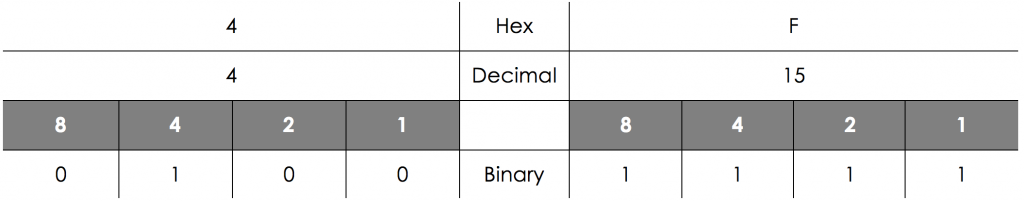So 4F in binary is 0100 1111.

Since we only really have 2 hexadecimal digits to consider, this is slightly more straightforward than converting binary numbers to decimal, but uses the same principles.

2. If there are letters, convert them to their decimal equivalent.
3. Multiply the value by the place heading.

Example - E6 in Decimal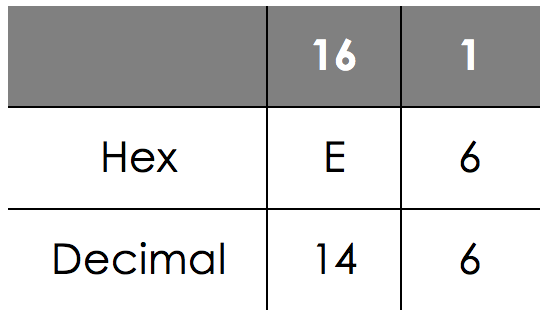So the decimal value would be:

(14 x 16) + (6 x 1) = 230

1. Work out how many 16s there are in the number
2. Write this number under the 16s column
3. Write the remainder under the 1s column
4. Covert these to hexadecimal digits (if applicable)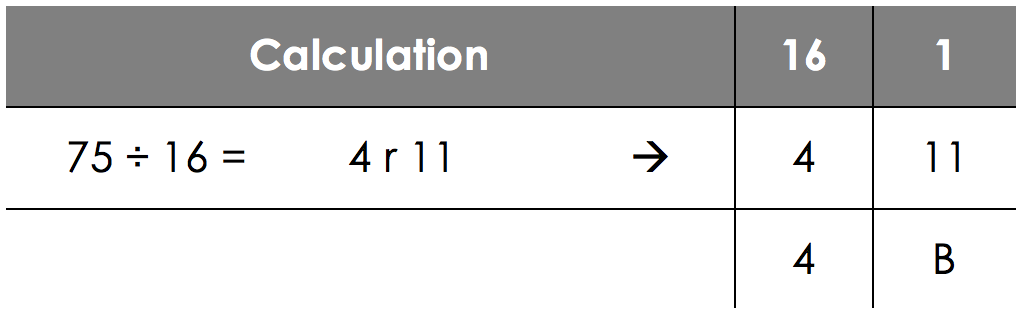So 75 in decimal is 4B in hexadecimal.

Convert these binary numbers to hexadecimal:0011 0101
35
Convert these binary numbers to hexadecimal:1100 0011
C3
Convert these binary numbers to hexadecimal:1101 1111
DF
Convert these hexadecimal numbers to binary:24
00100100
Convert these hexadecimal numbers to binary:A6
10100110
Convert these hexadecimal numbers to binary:CA
11001010
Convert these hexadecimal numbers to decimal:17
23
Convert these hexadecimal numbers to decimal:9A
154
Convert these hexadecimal numbers to decimal:F5
245
Convert these decimal numbers to hexadecimal:72
48
Convert these decimal numbers to hexadecimal:162
A2
Convert these decimal numbers to hexadecimal:241
F1